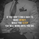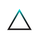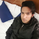# Transient Zones and Recurrent Zones by M.Uneeb2498 views
This is a Transient and Recurrent Zone Script. Study the following links to dig deeper in to this statistical idea:

• http://forex0k.blogspot.my/2015/09/forex-strategy-transient-zones.html
Protected script
This script is published closed-source and you may use it freely. You can favorite it to use it on a chart. You cannot view or modify its source code.
Want to use this script on a chart?M. Uneeb can you share the link for website for better understanding about your strategy. As the link you shared earlier is not working now. Regards.I tested it but it seems useless unfortunately. Why do the big arrows appear so late? They appear/ paint long after the moves have been made. Thanks thoughI will reveal the actual script of this Transient Zones and Recurrent Zones here in this comment:

//Start of code
//TZ and RZ percent occurrence by Muhammad Uneeb
study("Transient Zones and Recurrent Zones by M.Uneeb", "TZ and RZ by M.Uneeb", overlay=true)

//inputs
h_left = input(title="H left", type=integer, defval=10)
h_right = input(title="H right", type=integer, defval=10)
sample_period = input(title="Sample bars for % TZ", type=integer, defval=5000)
show_ptz = input(title="Show PTZ", type=bool, defval=true)
show_channel = input(title="Show channel", type=bool, defval=true)

//barCount = nz(barCount) + 1
//check history and realtime PTZ
h_left_low = lowest(h_left)
h_left_high = highest(h_left)
newlow = low <= h_left_low
newhigh = high >= h_left_high
plotshape(newlow and show_ptz, style=shape.triangledown, location=location.belowbar, color=red)
plotshape(newhigh and show_ptz, style=shape.triangleup, location=location.abovebar, color=green)
channel_high = plot(show_channel ? h_left_low : 0, color=silver)
channel_low = plot (show_channel ? h_left_high : 0, color=silver)

//check true TZ back in history
central_bar_low = low
central_bar_high = high
full_zone_low = lowest(h_left + h_right + 1)
full_zone_high = highest(h_left + h_right + 1)
central_bar_is_highest = central_bar_high >= full_zone_high
central_bar_is_lowest = central_bar_low <= full_zone_low
plotarrow(central_bar_is_highest ? -1 : 0, offset=-h_right-1)
plotarrow(central_bar_is_lowest ? 1 : 0, offset=-h_right-1)

//TZ probability calc
x = central_bar_is_highest ? 1 : 0
high_bar_tz_count = cum(x)

y = central_bar_is_lowest ? 1 : 0
low_bar_tz_count = cum(y)

total_tz = high_bar_tz_count + low_bar_tz_count
percent_tz_high = (high_bar_tz_count / sample_period) * 100
//plot(percent_tz_high, color = lime, transp=100)
percent_tz_low = (low_bar_tz_count / sample_period) * 100

//plot(low_bar_tz_count, color=red, transp=100)
percent_total_tz = (percent_tz_high + percent_tz_low)
plot(percent_total_tz, color=black, transp=100)

//PTZ probability calc
i = newhigh ? 1 : 0
high_bar_ptz_count = cum(i)

j = newlow ? 1 : 0
low_bar_ptz_count = cum(j)
total_ptz = high_bar_ptz_count + low_bar_ptz_count
percent_ptz_high = (high_bar_ptz_count / sample_period) * 100

//plot(percent_ptz_high, color=green, transp=100)
percent_ptz_low = (low_bar_ptz_count / sample_period) * 100

//plot(percent_ptz_low, color=maroon, transp=100)
percent_total_ptz = (percent_ptz_high + percent_ptz_low)
plot(percent_total_ptz, color=navy, transp=100)

//PTZ resolving probability calc
percent_ptz_resolved = (1 - (total_tz / total_ptz)) * 100
plot(percent_ptz_resolved, color=gray, transp=100)

//Median Channel
plot(show_channel ? (h_left_high + h_left_low) / 2 : 0, color=silver)

//MA 20
MA20 = interval > 1500 ? na : isintraday ? security(ticker, "1", sma(close,20)) : na
plot(MA20, color=blue, style=line, title="MA 20")

//MA 50
MA50 = interval > 1500 ? na : isintraday ? security(ticker, "1", sma(close,50)) : na
plot(MA50, color=red, style=line, title="MA 50")

//End of Code

You all can follow my website to learn how to apply this strategy in Binary Options Trading!
The relevant link of this particular strategy is as follows: